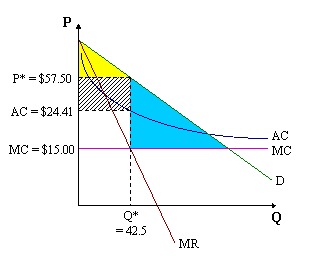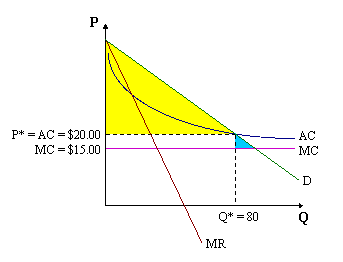Barry Haworth
University of Louisville
Department of Economics
Economics 201

### Natural Monopolies and Pricing Policy

Assume that a certain natural monopolist has the following demand and cost related curves:

 Demand: P = 100 - Q Marginal Revenue: MR = 100 - 2Q Average Cost: AC = 15 + (400/Q) Marginal Cost: MC = 15

Why is this a natural monopoly?
The answer stems from the monopolist's natural (cost-related) barriers to entry. The relative position of the AC and MC curves give the natural monopolist a cost advantage over its competition. Taking a closer look at these equations, you'll see that AC is always going to be greater than MC. Remembering the relationship between marginal and average values, AC will be declining as long as MC is below it. In general then, for a natural monopoly, AC is said to decrease (as Q increases) through "some relevant range of market output".

On a graph, it looks like this:We'll calculate the values for P* and Q* below, and also explain the meaning of the shaded areas.

If allowed to decide herself, how much will this natural monopolist produce, and at what price?
If allowed to set her own output and price, this natural monopolist will produce where MR = MC:

Set MR = MC, and solve for Q*

100 - 2Q = 15

Q* = 42.5

Find price by plugging Q* into the demand equation:

P = 100 - (42.5) = 57.5

Therefore: Q* = 42.5 and P* = \$57.50

Suppose we also want to find the monopolist's profits. To do that, we use the formula (P - AC)Q. Before plugging things into this equation though, we must find AC. The value for AC is found by plugging Q* into the AC equation to get AC = \$24.41 (i.e. AC = 15 + 400/42.5).

Profits are then calculated as:

p = [\$57.50 - \$24.41]42.5 = \$1406.33

To make these kind of profits (the area represented on the graph by the striped rectangle), the monopolist sets a price exceeding what might occur within a more competitive market. This high price makes consumer surplus (shaded yellow in the graph) rather small.

One big problem with this result is that since the natural monopolist produces less output than what is possible under perfect competition, there is some deadweight loss (shaded blue on the graph) -- which represents the value of output not produced as a result of P > MC.

To get rid of the DWL, a government regulator might step in and force the monopolist to set its price at marginal cost.

1. Marginal Cost pricing:
When the regulating agency forces this firm to set its price at marginal cost, we have what is called marginal cost pricing. In this case, that means setting P = \$15.

How much will the firm produce when P = MC?

Set Demand, or P, equal to MC and solve for Q*

100 - Q = 15

Q* = 85

What are the firm's profits when P = MC?

If P* = \$15 and Q* = 85, then AC = \$19.71
Profits become:

p = [\$15.00 - \$19.71]85 = -\$400.35

which represents a loss. On the graph below, these values and the areas for consumer surplus and profits are illustrated. Notice that the area of consumer surplus overlaps that corresponding with profit (loss), and that there is no deadweight loss since P = MC.Since the firm is making a loss, it needs to consider the future. That is, should the monopolist stay in this industry if, over the long run, the best it can ever do each year is make some type of loss? The answer would obviously be no, and so if the price were set at \$15, the firm would eventually exit the industry.

The whole point of government involvement here relates to the fact that regulators wanted to make things more efficient (in terms of allocative efficiency). However, achieving this particular type of efficiency causes the firm to eventually exit the industry -- leaving consumers with nothing. Therefore, to prevent the firm from leaving, our regulator must also allow the monopolist to cover her losses. One way to do this is by subsidizing the monopolist the amount of her loss (\$400.35). Another way is to give up on the idea of producing where P = MC.

2. Average Cost pricing:
One possibility is that the government regulator might want to allow the firm to charge a slightly higher price, but make zero economic profit. This is accomplished when P = AC, an approach that is called Average Cost pricing.

Figuring out (algebraically) what the price will be is a bit more involved than what we did above. To algebraically find the price that would equal average cost, we first set Demand (Price) equal to Average Cost (AC), then solve for Q* and lastly plug Q* into the Demand equation to get P*:

Set P = AC:

100 - Q = (400/Q) + 15

Rearrange this equation to get:

Q2 - 85Q + 400 = 0

Using the quadratic formula, we can solve for Q:

Q* = 80

Plugging Q* into the Demand equation, we can solve for P*

P* = 100 - (80) = \$20

Consequently, setting P = AC means setting P* = \$20, and getting 80 units of output. In the graph below, these values are given, as are the corresponding shaded areas for consumer surplus and deadweight loss (remember that profits are zero here since P = AC, but there will be some deadweight loss since P > MC).Of course, the problem here is that while the natural monopolist is able to make zero profits, thereby ensuring that the firm will stay in business, some deadweight loss reoccurs -- the very thing that government involvement was trying to eliminate.

Another potential problem with government imposing this type of (average cost) pricing is that it may create an incentive for the firm to inflate its fixed costs. This is called overcapitalization, because the firm may overinvest in capital equipment since it is, in a sense, guaranteed a "normal return".

What we find is that, when charging a single price to all consumers, a natural monopolist's costs force us to choose between allocative efficiency and allowing the firm a fair return on its investment (without subsidizing it). A final approach involves using two different "prices", what we'll call here a two part tariff.

3. Two-part tariff:
Suppose the regulator forces our monopolist to sell every unit of output at \$15 (i.e. P = MC), but also allows her to charge a fixed (flat) fee that all consumers must pay before buying this product at \$15.

In other words, the natural monopoly is allowed to charge something we could call an admittance fee. This fee establishes who is in the market. Those consumers who pay the fee are subsequently allowed to buy as much product as they want at \$15 per unit (the MC price).

Before this extra fee, a price of \$15 caused the monopolist to lose \$400 in profits. But with it, the fixed fee allows the monopolist to recoup those losses (by setting the fixed fee = 400/N, where N is the number of consumers who want to purchase the firm's product).

For example, if there are 50 consumers who want to buy this natural monopolist's product, then the firm should be allowed to charge a flat fee of \$8. Doing so, allows the firm to produce 85 units of output and make zero economic profit. This means that the government regulators get what they want -- no deadweight loss -- and the firm gets what she wants -- a fair return on her investment (which is what we interpret zero economic profit to imply).